# Excel/WPS条件格式中如何使用公式?

280 次浏览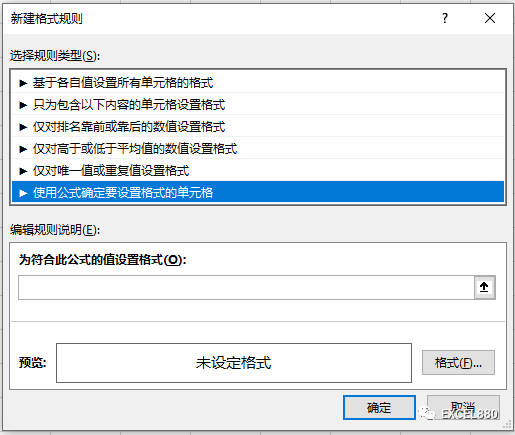• 公式的运算结果只能是TRUE或FALSE.
• TRUE执行条件格式，FALSE不执行。
• 条件格式的执行范围取决于两点：框选的范围；公式的锁定$1.与下拉菜单互动 =$A2=$F$2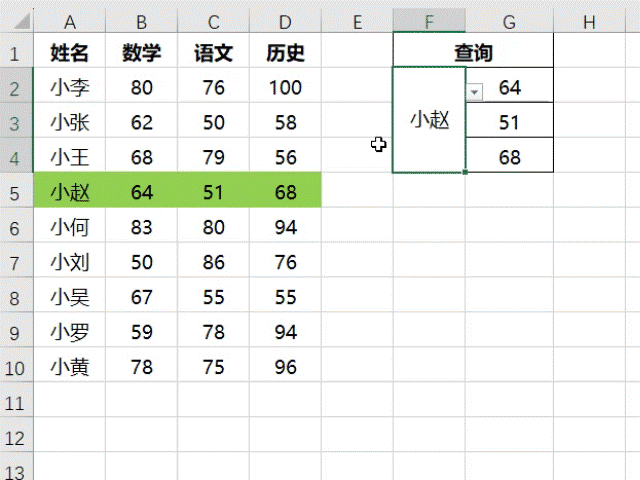2.突出显示有公式的单元格

=ISFORMULA(B2)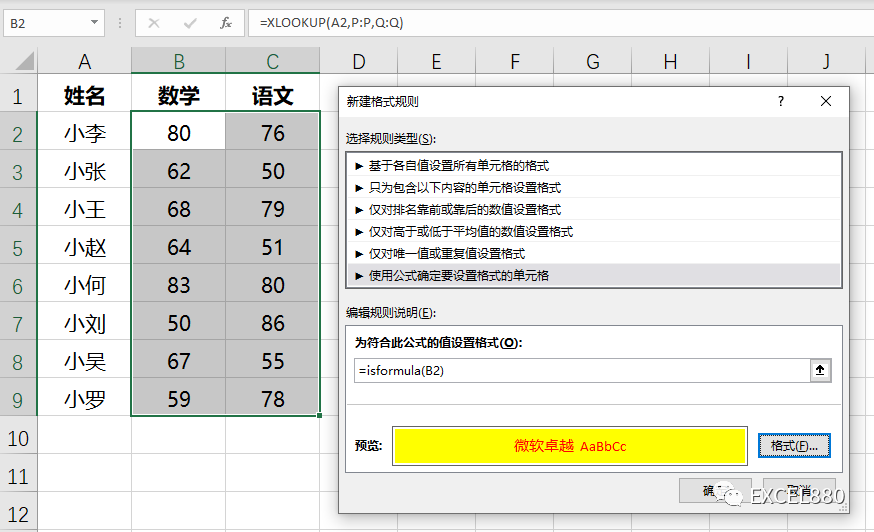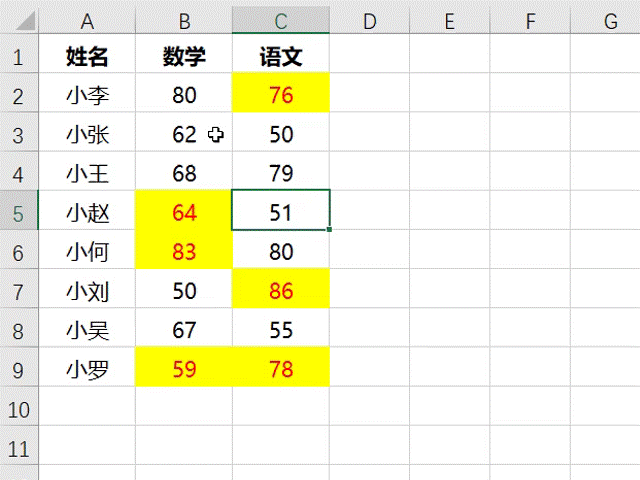3.自动添加边框

=\$A1<>””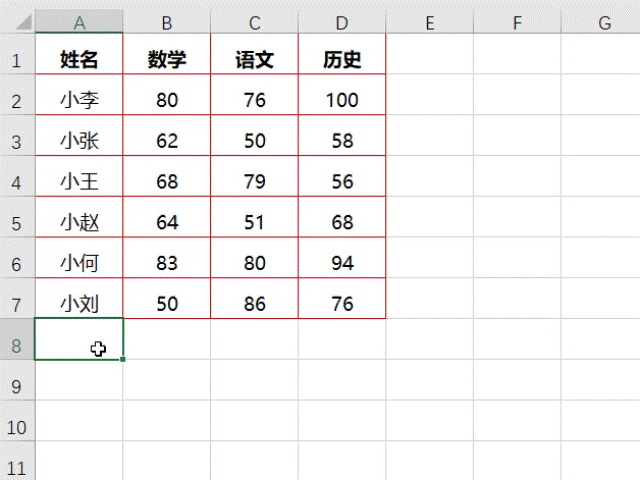4.根据日期判断周末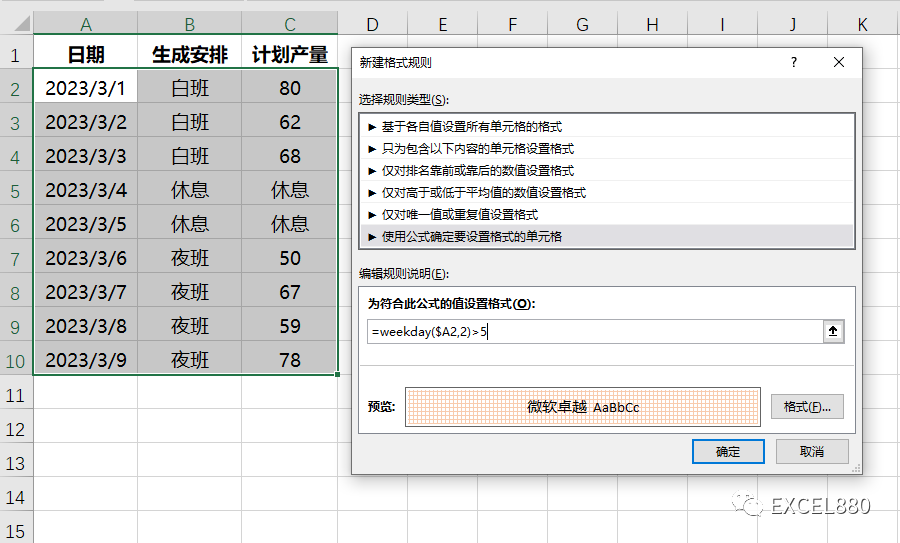（捣鼓半天设置出来的格式还是有点丑，你们搞个好看的吧！）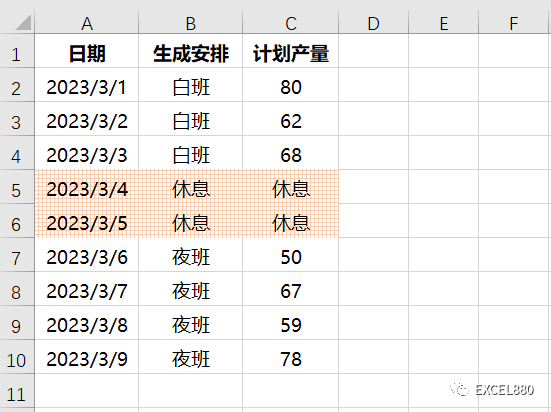5.找出有空格符的单元格

=FIND(” “,A1)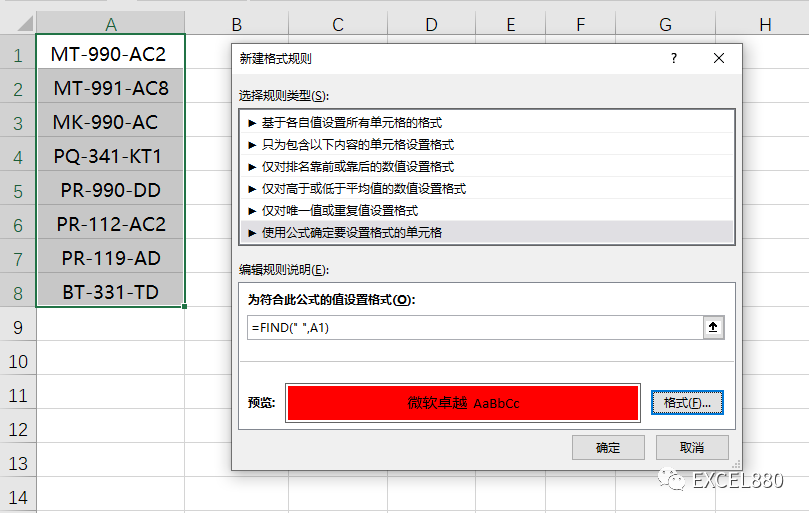Excel笔记：不等于0的数字可以被当作TRUE,错误值也可以被当作FASLE。

6.自动给离职人员添加删除线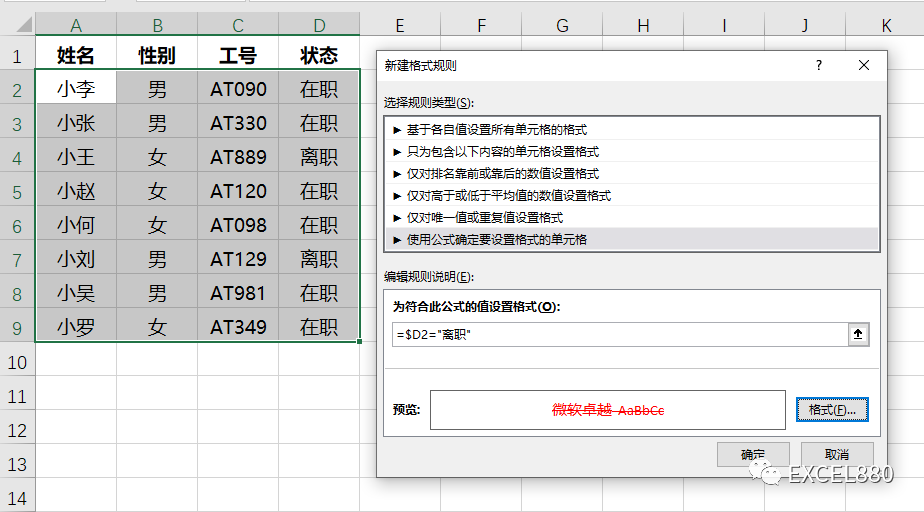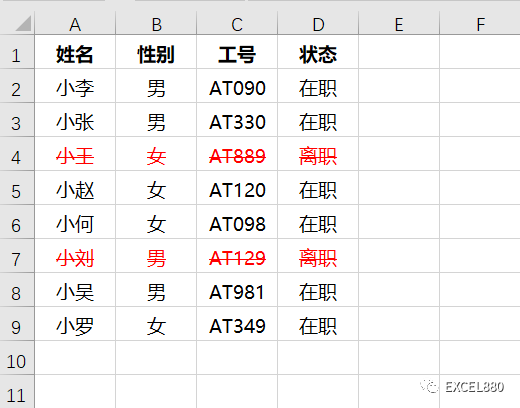7.标记电话号码不为11位的单元格

=LEN(A2)<>11

8.按行号突出显示

=MOD(ROW(1:1),2)

=ISODD(ROW(1:1))